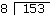Tuesday , 27 September 2022

# Mathematical Signs & Symbols Meanings

#### Mathematical Signs, Symbols and Definitions

 = Plus: sign addition 3 + 4 – Minus: sign of subtraction 4 – 2 x Multiplication: sign of multiplication: 4 X 2 ÷ Division sign: 8 ÷ 2 = Equals: 2 + 3 = 9 – 4 ≠ Not equal to: 2 + 3 = 9 – 6 > Greater than: 8 > 4 or 8 is greater than 4 < Less than: 4 < 8 or 4 is less than 8 ∞ Infinity: greater than any number we can write, speak or think ° Degree: unit of measurement for an angle; a complete circle has 360° π Pi: used to calculate the circumference and area of a circle; it equals 3.14159 ‘ Foot: used when measuring distance “ Inch: used when measuring distance ‘ Minute: used to measure parts of a degree; there are 60′ in 1° “ Second: used to measuring parts of a minute; there are 60″ in 1′ ⊥ Perpendicular to: forms a right angle || Parallel to: continues in a straight line and never meets

#### What is the language of division?

 19 Quotient: result of the division. Divisor: number by which dividend is to be dividedDividend: number to be divided by the divisor. 8 73 72 1 Reminder: number left over at the end of the division if the divisor doesnot divide the dividend evenly.

#### What is the language of fractions?

 4 Numerator: Indicates number of parts used. — 9 Denominator: Term below the line that divides the numerator; number of parts into which the whole is divided. 6 Proper Fraction: Numerator is smaller than denominator. — 7 13 Improper Fraction: Numerator is larger than denominator. — 4 2 ¼ Mixed Number: A whole number and a fraction.

#### What are square roots, radicals and exponents?

 √ Radical indicates the root of a number. It is usuallyused to indicate square root. For example, √4 = 2, since 2 x 2 = 4. A square root of any number is another number, which, when multiplied by itself, equals that number. Here is another example: √16 = ? The answer is 4, since 4 x 4 = 16. 3√ When the radical is used with a small number in the upper left, it stands for a smaller root. For example 3√27 = 3, since 3 x 3 x 3 = 27. In the same way 4√256 stands for the fourth root of 256, or a number multiplied by itself four times to equal 256. The answer is 4, since 4 x 4 x 4 x 4 = 256. A small number written at the upper right hand corner of another number is called an exponent. It is mathematical shorthand to indicate the number of times you have to multiply the number by itself. For example: 2² = 2 x 2 or 4. If we wrote 3³ we would mean 3 x 3 x 3, or 3 multiplied by itself three times.

## World Tourism Day Information (27 Sept)

Since 1980, the United Nations World Tourism Organization has celebrated World Tourism Day (WTD) as …